Next: 2.6 Newton Methods Up: 2. Finite Element Method Previous: 2.4 Time Dependent Problems

# 2.5 Finite Element Spaces and Meshes

The basic concepts of Galerkin's method, such as weak formulation of the problem and the transformation of this weak formulation from the functional space with infinite basis into the functional space with finite basis, as presented in Sections 2.3 and Sections 2.4, are presented here to introduce the finite element method.

The construction of the finite element space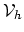begins with subdividing the domain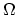into a set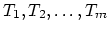of non-overlapping elements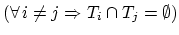. The domaincan now be approximated with a mesh domain,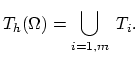(2.21)

We denote as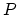the set of all points of the mesh domain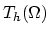. Each point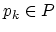has an unique global index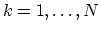, where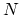is the number of all points in the mesh. A point has several local indices.

The basis functions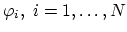of the finite element spacefulfill,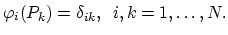(2.22)

Since they are defined on nodes of the mesh, we call them basis nodal functions. In the finite element praxis basis nodal functions are almost exclusively low order polynomials.

For further discussion it will be useful to have approximations of functions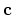represented for each element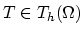with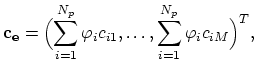(2.23)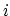represents the local index of the element vertices and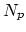is the number of element vertices.
We use linear basis functions and tethraedronsas elements. Therefore, and each of the functions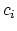,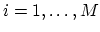is approximated on the elements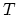of the discretizationusing the linear basis functions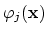,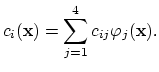(2.24)

Now we can also define the operator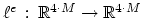for each element of the discretization,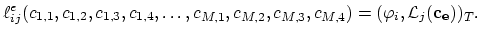(2.25)

The inner product is calculated over the element. The local residuum vector is defined as,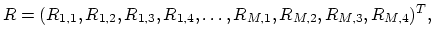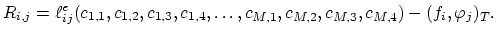(2.26)

Determining the operator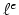requires the calculation of the basic nodal functions,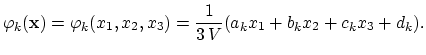(2.27)

The coefficients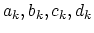are functions of the nodal coordinates and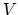is the volume of the element. Constructing offrequently demands calculations of the following integral,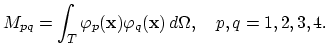(2.28)

In this case (2.27) can be used, but instead it is more practical to project the discretization element(Figure 2.1) into normalized coordinate system element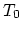(Figure 2.2). Each point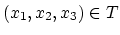is a bijective projection of the corresponding point,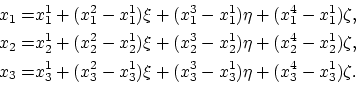(2.29)

The basis nodal functions onare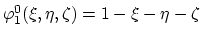,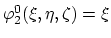,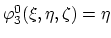and. (2.28) is now calculated as(2.30)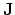is the Jacobian of the projection (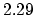),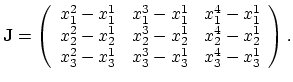(2.31)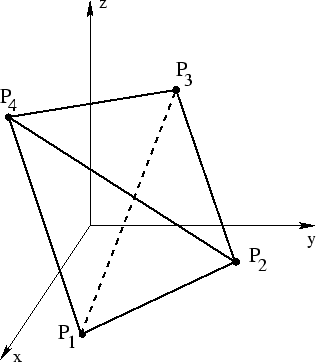Next: 2.6 Newton Methods Up: 2. Finite Element Method Previous: 2.4 Time Dependent Problems

H. Ceric: Numerical Techniques in Modern TCAD